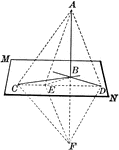### Line Perpendicular to Plane

If a straight line is perpendicular to each of two other straight lines in a plane at their point of…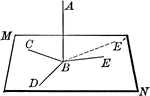### Line Perpendicular to Plane

Straight line AB is perpendicular to the lines in plane MN at point B.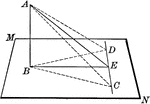### Line Perpendicular to Plane

Straight line AB is perpendicular to the lines in plane MN at point B. "If from the foot of a perpendicular…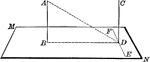### Lines Perpendicular to Plane

Two straight lines, AB and CD, perpendicular to the same plane (MN) are parallel.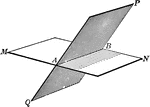### Intersecting Planes

The intersection of two planes is a straight line.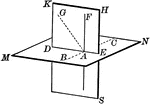### Intersecting Planes

The intersection of two planes is a straight line.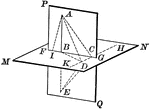### Intersecting Planes

The intersection of two planes is a straight line.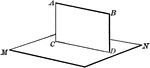### Intersecting Planes

The intersection of two planes is a straight line.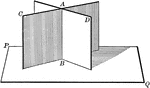### Intersecting Planes

Two intersecting planes on a third plane. "If two intersecting planes are each perpendicular to a third…